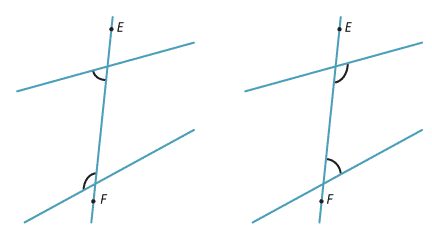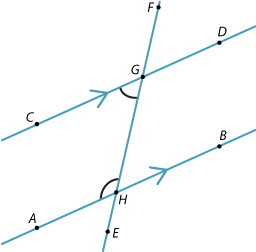Co-interior angles

In each diagram below, the two marked angles are co-interior angles because they are between the two lines and on the same side as the transversal EF.Co-interior angles and parallel lines

If the lines are parallel, then the sum of the co-interior angles is 180°.

They are supplementary angles.

This can be proven using the earlier results.$$\angle$$AHF = $$\angle$$EGD (alternate angles AB || CD)

$$\angle$$CGF + $$\angle$$EGD = 180° (straight angle at G)

Hence $$\angle$$AHF + $$\angle$$CGF = 180°.

Example 3

Find the angle θ in the diagram.Solution

θ = 180° – 95° (co-interior angles AB || CD) = 85°